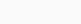# Brainly – Homework Math Solver# Brainly – Homework Math Solver

Rate : 26K+
v by Brainly

Quick Math Helper & Science Solutions | Get Homework Answers for School Doubts

Recommended posts
App Name Brainly – Homework Math Solver co.brainly Brainly Education 88.11 MB 100,000,000+ Dec 14, 2022Brainly is a powerful Math solver app that can help you with your school doubts. Solve Math problems in Algebra, Trigonometry, & Geometry with correct & expert-verified answers instantly. With Brainly, you can find solutions to your math homework. Math answers have never been easier to find! Just use our math scanner, ask our community, or chat with a tutor for one-on-one help with step-by-step solutions for your math homework problems.

• 24/7 school homework app access – Unlimited access, anywhere, anytime with Brainly.
• Quick math problem solver – Math Questions are answered in minutes
• Expert-vetted answers – A team of subject experts moderators verify answers daily
• Ask by pic with math camera scanner feature
• Solve Math Problems by using Brainly Scan to Solve: learn math with step-by-step guidance on Algebra, Trigonometry, Geometry, & Calculus, including AP levels
• Find Math answers specific solutions created by our experts for your textbooks.
• Real-time help from pro math tutors with Brainly Tutor (Math, Physics, Chemistry, & Biology tutors)

# Math Answer Scanner To Solve Math Problems

Do you ever wish you had a math helper? With Brainly, you'll get help instantly. Use our math scanner & word problem solver to find answers to complex problems. Brainly can work like a fraction calculator, a geometry solver, & an algebra practice tool. It’s like having a math helper in your pocket!
Deal with math concepts, & get instant math answers so you can say, “I’m done with my homework!” So, if you're stuck on a math problem, just use our math calculator as a problem solver.

# Get Homework Help from a Community of Experts

With over 350M online study buddies ready to help, you won’t need a homework reminder to find the motivation to learn. Our community of helpful student experts can get your math homework answers in minutes.
It's like having millions of math word problem solvers all at once! All your questions deserve an answer! Our network of moderators ensures not only high-quality homework help but also makes sure that Brainly remains a safe place for learning & math practice.
With Brainly, you can turn learning into a fun challenge, show off your achievements & earn cool badges for answering the trickiest questions.

# Get 1:1 Homework Help with Brainly Plus or Tutor

Sometimes, even a math calculator problem solver is not enough. If you're hungry for more quick math answers, you can upgrade to Brainly Tutor for Math, Physics, Chemistry, & Biology answers. With Brainly Tutor, you can instantly get connected to an expert to help you with your math problems.

With Brainly Plus, you can use our math solver without any limits or ads.

With Brainly, You are Learning Your Way! No matter what your math homework struggles are, we’re here to help you get unstuck in the easiest way possible.

Get fun updates from us on: